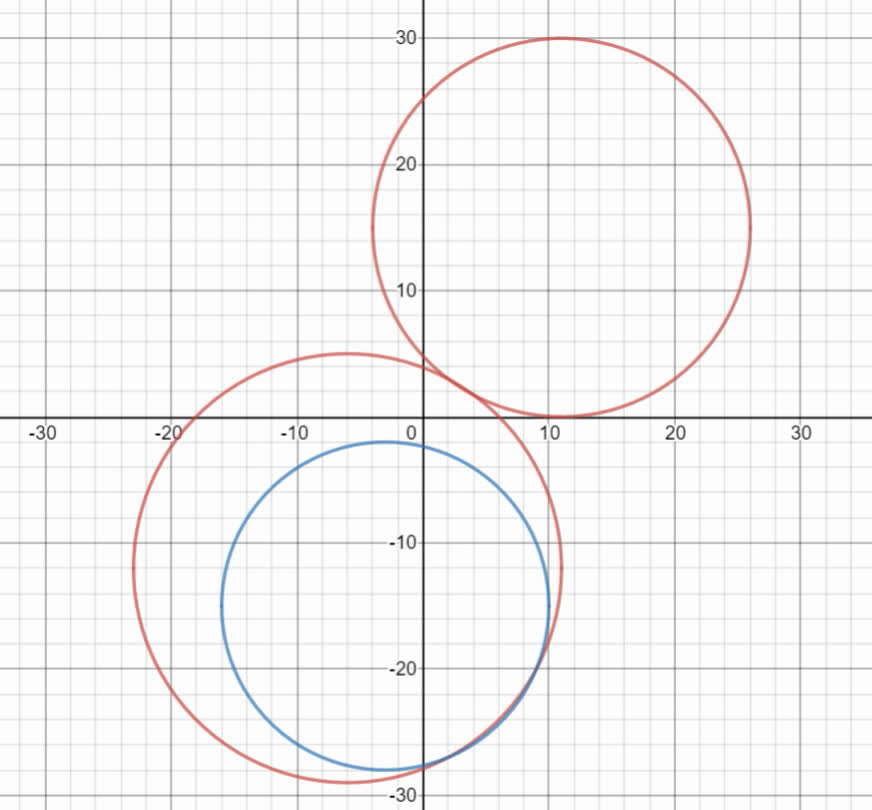# Three fire observation towers are located at points A (-6,-12), B (11,ka1leE 2021-08-18 Answered
Three fire observation towers are located at points A (-6,-12), B (11,15), and C (-3,15), on a map where all the units are in kilometers. A fire is located at distances of 17 km, 15km, and 13km, respectively, from the observation towers. Graph three center circles whose centers are located at the observation towers and whose radii are the given distances to the fire. Then estimate the location of the fire.

• Questions are typically answered in as fast as 30 minutes

### Plainmath recommends

• Get a detailed answer even on the hardest topics.
• Ask an expert for a step-by-step guidance to learn to do it yourself.Anonym

Equation for these three towers are:
$$\displaystyle{\left({x}+{6}\right)}^{{{2}}}+{\left({y}+{12}\right)}^{{{2}}}={17}^{{{2}}}$$
$$\displaystyle{\left({x}-{11}\right)}^{{{2}}}+{\left({y}-{15}\right)}^{{{2}}}={15}^{{{2}}}$$
$$\displaystyle{\left({x}+{3}\right)}^{{{2}}}+{\left({y}-{15}\right)}^{{{2}}}={13}^{{{2}}}$$